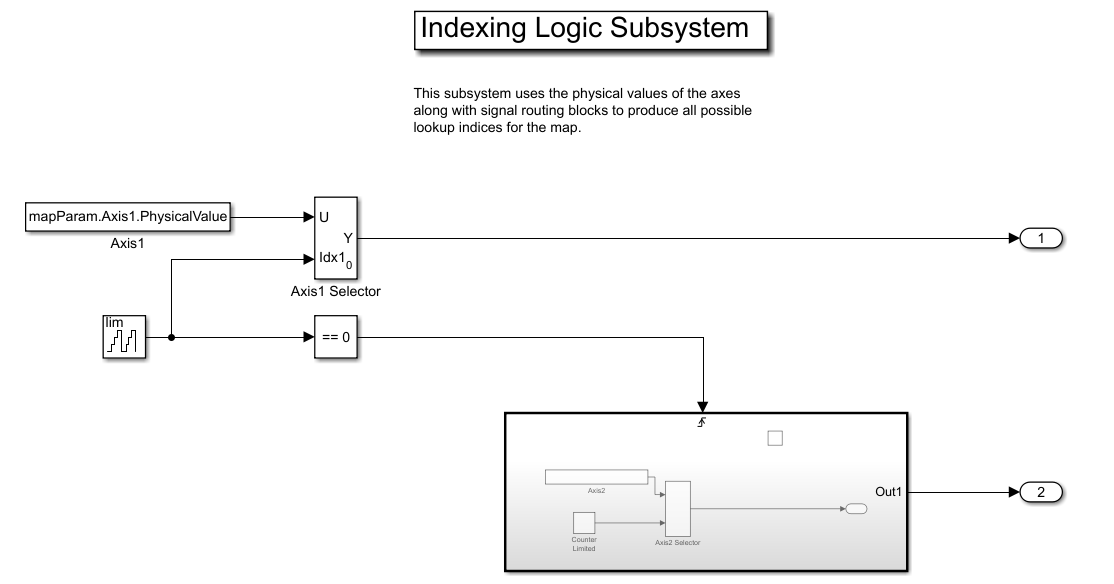This example shows how to use calibration data from a CDFX-file as inputs to a Simulink model.

Import Data

Import the calibration data using the cdfx function.

cdfxObj = cdfx("CDFXExampleFile.cdfx")
cdfxObj =
CDFX with properties:

Name: "CDFXExampleFile.cdfx"
Version: "CDF20"

Instantiate Local Variables

Use getValue to extract the desired parameters into the MATLAB workspace.

gainParam = getValue(cdfxObj, "ASAM.C.SCALAR.GAIN")
gainParam = 3
mapParam = getValue(cdfxObj, "ASAM.C.MAP")
mapParam = struct with fields:
PhysicalValue: [5x5 double]
Axis1: [1x1 struct]
Axis2: [1x1 struct]

Lookup-Gain Model

cdfxMdl = gcs
cdfxMdl =This model contains:

• 2-D Lookup Table block to represent the ASAM.C.MAP parameter from the CDFX-file. The "Table data" field represents the physical value of the instance, and the "Breakpoint" fields represent the physical values of the axes.

• Gain block to represent the ASAM.C.SCALAR.GAIN parameter from the CDFX-file.

• To Workspace block to log the simulation data.

Indexing Logic Subsystem

The Indexing Logic subsystem uses the physical values of the axes of the ASAM.C.MAP parameter, along with signal routing blocks and a triggered subsystem, to produce all valid combinations of lookup indices. This configuration can be useful if you need to test across the full range of possible input values of a calibration parameter.Log Output Data in MATLAB

The output of the simulation is sent to MATLAB by the To Workspace block, where it is stored as a timeseries object called mapData. This data can now be inspected and visualized in the MATLAB workspace.

sim(cdfxMdl);
plot(mapData)
title("Simulation Output for ASAM.C.MAP")% Copyright 2018-2021 The MathWorks, Inc.

Vehicle Network Toolbox DocumentationGet trial now